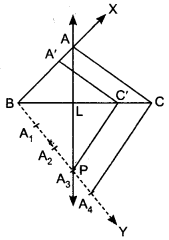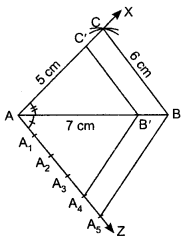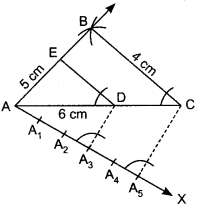## Extra Questions of Class 10 Maths Chapter 11 Constructions PDF Download

We have provided you with Extra and Important Questions from Class 10 Maths Chapter 11 Constructions .This Extra and Important Questions will help you to score 100% in your Board Exams. These extra questions will be helpful to revise the important topics and concepts.

# Constructions  Class 10 Important Questions with Answers Maths Chapter 11

## Extra Questions for Class 10 Maths Chapter 11 Very Short Answer Type

Question:Is construction of a triangle with sides 8 cm, 4 cm, 4 cm possible?

Solution:

No, we know that in a triangle sum of two sides of a triangle is greater than the third side. So the condition is not satisfied.

Question:To divide the line segment AB in the ratio 5 : 6, draw a ray AX such that ∠BAX is an acute angle, then draw a ray BY parallel to AX and the point A1, A2, A3… and B1, B2, B3… are located at equal distances on ray AX and BY respectively. Then which points should be joined?

Solution:

A5 and B6.

Question:To draw a pair of tangents to a circle which are inclined to each other at an angle of 60°, it is required to draw tangents at end points of those two radii of the circle. What should be the angle between them?

Solution:

120°

Question:In Fig. 9.1 by what ratio does P divide AB internally.

Solution:

From Fig. 9.1, it is clear that there are 3 points at equal distances on AX and 4 points at equal distances on BY. Here P divides AB on joining A3 B4. So P divides internally by 3 : 4.

## Extra Questions for Class 10 Maths Chapter 11 Short Answer Type

Question:

Construct a triangle of sides 4 cm, 5 cm and 6 cm and then a triangle similar to it whose sides are 23 of the corresponding sides of the first triangle.
OR
Draw a triangle with sides 4 cm, 5 cm and 6 cm. Then construct another triangle whose sides are 23 of the corresponding sides of first triangle.

Solution:

Steps of Construction:
Step I: Draw a line segment BC = 6 cm
Step II: Draw an arc with B as centre and radius equal to 5 cm.
Step III: Draw an arc, with C as centre and radius equal to 4 cm intersecting the previous drawn arc at A.
Step IV: Join AB and AC, then ∆ABC is the required triangle.
Step V: Below BC make an acute angle CBX
Step VI: Along BX mark off three points at equal distance: B1, B2, B3, such that BB1 = B1B2, = B2B3.
Step VII: Join BC3.
Step VIII: From B2, draw B2, D || B3,C, meeting BC at D.
Step IX: From D draw ED || AC meeting BA at E. Then we have ∆EDB which is the required triangle.Justification:
Since DE || CAHence, we have the new AEBD similar to the given ∆ABC, whose sides are equal to 23 of the corresponding sides of ∆ABC.

Question:Draw a line segment of length 7.6 cm and divide it in the ratio 5 : 8. Measure the two parts.

Solution:

Steps of Construction:
Step I: Draw a line segment AB = 7.6 cm
Step II: Draw any ray AX making an acute angle ∠BAX with AB.
Step III: On ray AX starting from A, mark 5 + 8 = 13 equal arcs. AA1, A1A2, A2A3, A3A4, … A11A12, and A12A13.
Step IV: Join A13B.
Step V: From A5, draw A5P || A13B, meeting AB at P. Thus, P divides AB in the ratio 5 : 8. On measuring the two parts. We find AP = 2.9 cm and PB = 4.7 cm (approx).

Justification:
In ∆ABA13, PA5 || BA13 .
∴ By Basic Proportionality Theorem

Question:Draw a circle of radius 6 cm. From a point 10 cm away from its centre, construct the pair of tangents to the circle and measure their lengths.

Solution:

Steps of Construction:
Step 1: Take a point O and draw a circle of radius 6 cm.
Step II: Take a point P at a distance of 10 cm from the centre 0.
Step III: Join OP and bisect it. Let M be the mid-point.
Step IV: With M as centre and MP as radius, draw a circle to intersect the circle at Q and R.
Step V: Join PQ and PR. Then, PQ and PR are the required tangents. On measuring, we find, PQ = PR = 8cm.

Justification:
On joining OQ, we find that ∠PQO = 90°, as ∠PQO is the angle in the Semicircle.
∴ PQ ⊥ OQ
Since OQ is the radius of the given circle, PQ has to be a tangent to the circle. Similarly, PR is
also a tangent to the circle

Question:Construct a tangent to a circle of radius 4 cm from a point on the concentric circle of radius 6 cm and measure its length. Also, verify the measurement by actual calculation.

Solution:

Steps of Construction:
Step 1: Take a point O and draw a circle of radius OA = 4 cm. Also, draw a concentric circle of radius OB = 6 cm
Step II: Find the mid-point C of OB and draw a circle of radius OC = BC. Suppose this circle intersects the circle of radius 4 cm at P and Q.
Step III: Join BP and BQ to get the desired tangents from a point B on the circle of radius 6 cm. By actual measurement, we find BP = BQ = 4.5 cm.

Justification:
In ∆BPO, we have
∠BPO = 90°, OB = 6 cm and OP = 4 cm
∴ OB2 = BP2 + OP2 [Using Pythagoras theorem]

Similarly, BQ = 4.47 cm

Question:Draw a line segment AB of length 8 cm. Taking A as centre, draw a circle of radius 4 cm and
taking B as centre, draw another circle of radius 3 cm. Construct tangents to each circle from the centre of the other circle.

Solution:

Steps of Construction:
Step I: Draw a line segment AB = 8 cm.
Step II: With A as centre, draw a circle of radius 4 cm and let it intersect the line segment AB in M.
Step III: With B as centre, draw a circle of radius 3 cm.
Step IV: With M as centre, draw a circle of radius AM and let it intersect the given two circles in P, e and R, S.
Step V: Join AP, AQ, BR and BS.
These are the required tangents.

Justification:
On joining BP, we have ∠BPA = 90°, as ∠BPA is the angle in the semicircle.
∴ AP ⊥ PB
Since BP is the radius of given circle, so AP has to be a tangent to the circle. Similarly, AQ, BR and BS are the tangents.

Question:Draw a circle of radius of 3 cm. Take two points P and Q on one of its diameters extended on both sides, each at a distance of 7 cm on opposite sides of its centre. Draw tangents to the circle from these two points P and Q.

Solution:

Steps of Construction:
Step 1: Taking a point ( as centre, draw a circle of radius 3 cm.
Step II: Take two points P and Q on one of its extended diameter such that OP = OQ = 7 cm.
Step III: Bisect OP and OQ and let M1 and M2 be the mid-points of OP and OQ respectively.
Step IV: Draw a circle with M1 as centre and M1 P as radius to intersect the circle at T1, and T2.
Step V: Join PT1 and PT2.
Then, PT1 and PT2 are the required tangents. Similarly, the tangents QT3 and QT4 can be obtained

Justification:
On joining OT1, we find ∠PT1O = 90°, as it is an angle in the semicircle.
PT1 ⊥ OT1
Since OT1 is a radius of the given circle, so PT1 has to be a tangent to the circle.
Similarly, PT2, QT3 and QT4 are also tangents to the circle.

Question:Let ABC be a right triangle in which AB = 6 cm, BC = 8 cm and ∠B = 90°. BD is the perpendicular from B on AC. The circle through B, C, D is drawn. Construct the tangents from A to this circle.

Solution:

Steps of Construction:
Step I: Draw ∆ABC and perpendicular BD from B on AC.
Step II: Draw a circle with BC as a diameter. This circle will pass through D.
Step III: Let O be the mid-point of BC. Join A0.
Step IV: Draw a circle with AO as diameter. This circle cuts the circle drawn in step II at B and E.
Step V: Join AE. AE and AB are desired tangents drawn from A to the circle passing through B, C and D.

## Extra Questions for Class 10 Maths Chapter 11 Long Answer Type

Question:Draw a circle with the help of a bangle. Take a point outside the circle. Construct the pair of tangents from this point to the circle.

Solution:Steps of Construction:
Step I: Draw a circle with the help of a bangle.
Step II: Let P be the external point from where the tangents are to be drawn to the given circle. Through P, draw a secant PAB to intersect the circle at A and B (say).
Step III: Produce AP to a point C, such that AP = PC, i.e., P, is the mid-point of AC.
Step IV: Draw a semicircle with BC as diameter.
Step V: Draw PD ⊥ CB, intersecting the semicircle at D.
Step VI: With P as centre and PD as radius, draw arcs to intersect the given circle at T and T1.
Step VII: Join PT and PT1. Then, PT and PT1 are the required tangents.

Question:Draw a pair of tangents to a circle of radius 4 cm which are inclined to each other at an angle of 60°
OR
Draw a circle of radius 4 cm. Construct a pair of tangents to it, the angle between which is 60°. Also justify the construction. Measure the distance between the centre of the circle and the point of intersection of tangents.

Solution:

Steps of Construction:
Step I: Draw a circle with centre 0 and radius 4 cm.
Step II: Draw any diameter AOB.
Step III: Draw a radius OC such that ∠BOC = 60°.
Step IV: At C, we draw CM ⊥ OC and at A, we draw AN ⊥ OA.
Step V: Let the two perpendiculars intersect each other at P. Then, PA and PC are required tangents.

Justification:
Since OA is the radius, so PA has to be a tangent to the circle. Similarly, PC is also tangent to the circle.
∠APC = 360° – (∠OAP + ∠OCP + ∠AOC)
= 360° – (90° + 90° + 120°) = 360° – 300° = 60°
Hence, tangents PA and PC are inclined to each other at an angle of 60°

Question:Draw an isosceles ΔABC in which BC = 5.5 cm and altitude AL = 3 cm. Then construct another triangle whose sides are  3/4 of the corresponding sides of ΔABC

Solution:Steps of construction:

1. Draw BC = 5.5 cm.
2. Construct AP the perpendicular bisector of BC meeting BC at L.
3. Along LP cut off LA = 3 cm.
4. Join BA and CA. Then ΔABC so obtained is the required ΔABC.
5. Draw an acute angle CBY and cut 4 equal lengths as BA1 = A1A2 = A2A3 = A3A4 and join CA4.
6. Now draw a line through A3 parallel to CA4 intersecting BC at C’.
7. Draw a line through C’ and parallel to AC intersecting AB at A’. BA’C’ is the required triangle.

Question:Draw a triangle with sides 5 cm, 6 cm and 7 cm. Then draw another triangle whose 4/5 sides are y of the corresponding sides of first triangle

Solution:1. Draw a line segment AB of length 7 cm.
Then using A as centre and distance 5 cm draw an arc C.
Also draw an arc using B as centre and with distance 6 cm, which intersect earlier drawn arc at C. Join AC and BC.
2. Draw an acute angle BAZ and cut AZ as AA1 = A1A2 = A2A3 = A3A4 = A4A5 and join BA5.
3. Through A4 draw a line parallel BA5 intersecting AB at B’.
4. Through B’ draw a line parallel to BC intersecting AC at C’. AAB’C’ is the required triangle.

Question:Draw a ΔABC in which AB = 4 cm, BC = 5 cm and AC = 6 cm. Then construct another triangle whose sides are 3/5 of the corresponding sides of ΔABC

Solution:Steps of construction:

1. Draw a line segment AC = 6 cm.
2. Draw an arc with A as centre and radius equal to 5 cm.
3. Draw an arc with C as centre and radius equal to 4 cm intersecting the previous drawn arc at B.
4. Join AB and CB, then ΔABC is required triangle.
5. Below AC make an acute angle CAX.
6. Along AX mark of 5 points A1, A2, A3, A4, A5 such that AA1= A1A2 = A2A3= A3A4 = A4A5.
7. Join A5C.
8. From A3 draw A3D | | A3C meeting AC at D.
9. From D, draw ED | | BC meeting AB at E. Then we have ΔEDA which is the required triangle.